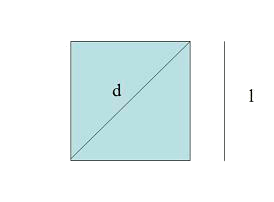# The squareThe area of the square is: $$A=l^2$$$Its perimeter is: $$P=4 \cdot l$$$

And, applying the Pythagorean theorem, its diagonal is: $$d=\sqrt{2 \cdot l^2}= l\cdot \sqrt{2}$$$Calculate the area of a square which diagonal is $$3$$ cm. • First find the side of the square: $$3^2=l^2+l^2=2 \cdot l^2$$$ $$l^2=\frac{9}{2}$$$$$l=\frac{3}{\sqrt{2}} \ cm$$$

• Let's calculate the area:

$$A=\Big( \frac{3}{\sqrt{2}}\Big)^2=\frac{9}{2} \ cm^2$$\$